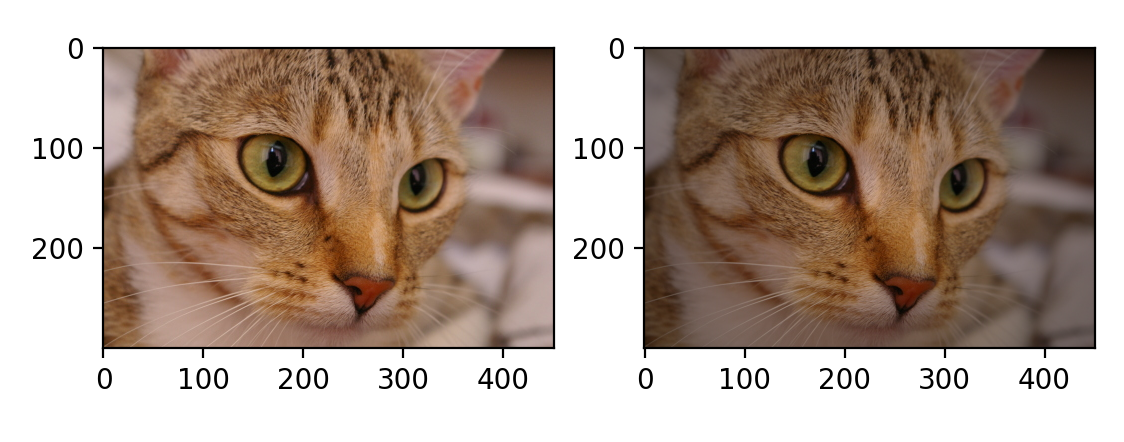# Implementing a Vignette

I recently had a task to create a vignette of a picture. This is a technique in Computer Vision or digital signal processing whereby as you move closer and closer towards the centre of the image, the pixel intensity increases:When first approaching this problem, I could not understand how, from a dense matrix of colour information, you could determine how far the pixel that you were processing was from the centre of the image. I confirmed, that no position information is available within the pixel itself (it just contains raw colour information). Finally, it dawned on me that you could use the coordinates of the image matrix to create a vector to represent the centre point, ie. the offset x and y from an origin.

But where is the origin?

I first thought I'd have to use the centre of the image as the origin, meaning all my pixels co-ordinates would need to be relative to that, which would have been a pain as I'd have to work out how, say each pixel in the matrix[x][y] would probably need to be represented differently when relative to not matrix but the centre of the image!

Then I realised that it could keep the origin as  in the matrix ie image[0,0] for both the centre point and each respective pixel and then it could thus be represented by a vector displacement from that same origin. This was a breakthrough for me. Not only that, you could then generate a new vector for each pixel this way - all using [0,0] in the image matrix to represent a distance of that pixel from the same origin.

So, now you have two 2D vectors from the same origin, one that points at the centre of the image ie [max_cols/2, max_rows/2] and you have a vector that is [x,y] for each pixel you are currently processing. You can now subtract the vector representing the centre point from the pixel vector you are currently at, ie this would result in the vector between the two, which if you can calculate the magnitude thereof, will be the distance between the pixel you are on and the centre of the screen - ie its the hypotenuse between the two sides (of the two vectors).

The length of the resulting vector can be easily by passing in the vector to np.linalg.norm() - ie get the norm of the vector ie the (length or magnitude) and this the distance. I guess you could also do this yourself by squaring the components of the vectors, adding them up together and taking the square root. But this is much easier.

Now you can use that distance to drive the intensity value at that pixel!

With the distance(d), you can derive the relative intensity using a function of d and max length of the image to give the intensity i.e brightness(d,img_max) to assign for that distance from the centre. That function produces e raised to the power of the ratio of distance to width. This equation was given and I've represented as the brightness function in the python code below:

$f(x,y) = e^{(\frac{-x}{y})} = \begin{cases} & \text{x is distance from center} \\ & \text{y is the width of image } \end{cases}$

As the image was already in 24-bit RGB colour, I converted it to HSV so that I could manipulate the V component, which corresponds to the intensity. This is not immediately and easily determinable from the RGB data.

I could then manipulate the V component by doing a simple 1d vector x 1d vector multiplication to create a mask that multiplies only the V component with the brightness, leaving the Hue and Saturation intact (I multiplied those by 1(identity))

This can be more concisely be represented in this Python script I wrote:

# Change intensity of pixel colour, depending on the distance the pixel is from the centre of the image

from skimage import data
import numpy as np
import matplotlib.pyplot as plot
from skimage import color, img_as_float, img_as_ubyte
import math

# We can use the built in image of Chelsea the cat
cat = data.chelsea()

# Convert to HSV to be able to manipulate the image intensity(v)
cat_vig = color.rgb2hsv(cat.copy())

print(f'Data type of cat is: {cat_vig.dtype}')
print(f'Shape of cat is: {cat_vig.shape}')

# Get the dimension of the matrix (n-dimensional array of colour information)
[r, c, depth] = cat_vig.shape
v_center = [c / 2, r / 2, 0]

# Derive the pixel intensity from the distance from center

# Go through each pixel and calculate its distance from center
# feed this into the brightness function
# modify the intensity component (v) of [h,s,v] for that pixel
def version1(rows, cols, rgb_img, v_center):
for y in range(rows):
for x in range(cols):
me = np.array([x, y, 0])
dist = np.linalg.norm(v_center - me)
# alternative:
cat_vig[y][x] *= [1, 1, brightness(dist, cols)]
# cat_vign[y][x] *= brightness(dist, cols)

# do it
version1(r,c, cat_vig, v_center)

# Convert back to RGB so we can show in imshow()
cat_vig = color.hsv2rgb(cat_vig)
fig, ax = plot.subplots(1, 2)
ax.imshow(cat)  # Original version
ax.imshow(cat_vig)  # vignette version
plot.show()


This was pretty interesting, and I do love it when theoretical math ie linear algebra applies to practical outcomes!

Q.E.D: